# brain of mat kelcey...

## a half baked pix2pix experiment for road trip videos with teaching forcing

June 26, 2019 at 01:00 PM | categories: gan, projects

for the last few months i've been tinkering on/off with a half baked sequential pix2pix model. it's got a lot of TODOs to make it work but i've been distracted by something else and i'll feel less guilty about dropping it temporarily if i scrappily write a blog post at least...

for the last month we lived in the U.S. we drove around california, utah, nevada and colorado in a huge RV. occsionally i had a time lapse running on my phone which resulted in 16,000 or so stills.

 some random frames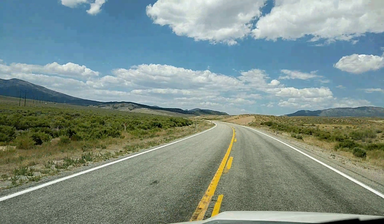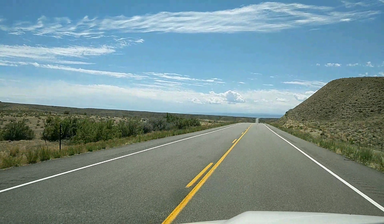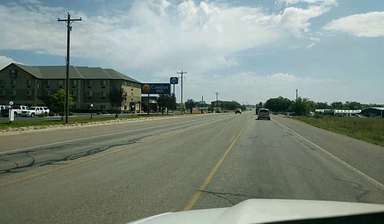to get a sense of the time between frames here are three sequential ones ...

 three sequential frames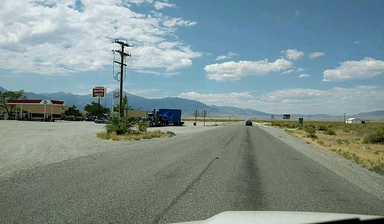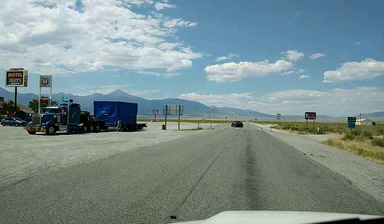can we train a $$next\_frame$$ model that given two frames predicts the next? if so, can we use it for generation by rolling out frames and feeding them back in.

( i saw an awesome example of this a few years ago when someone did this for view out the side of a train but can't find the reference now sadly :( if you know the project, please let me know! )

## v1 model

the simplest formulation is just $$\hat{f_3} = next\_frame(f_1, f_2)$$

we encode frames f1 and f2 using a shared encoder, concat the representations, do a little more encoding then decode back to an image. we just train on the L1 difference $$L1(f_3, \hat{f_3})$$completely unsurprisingly, given this loss, the model just learns a weird blurry middle ground for all possible frames. pretty standard for L1.

note: i test here on a particularly difficult sequence that has pretty rare features (other cars!!) to get some vague sense of how much it's memorising things...

 example rollout of v1 model## v2 model

when we train the v1 model, let's consider how we use it for rollouts;

• we start with two known frames, $$f_1, f_2$$
• we predict the third frame by running these through the model... $$\hat{f_3} = next\_frame(f_1, f_2)$$
• we predict the fourth frame by refeeding this back in... $$\hat{f_4} = next\_frame(f_2, \hat{f_3})$$

this fails for too reasons; the L1 loss as before just isn't that good but additionally in the last step we're asking the model to make a prediction about a frame after $$\hat{f_3}$$ and given $$\hat{f_3}$$ isn't that much like what it's seen before, the network produces an even worse output and the problems compound.

interestingly though for the $$\hat{f_4} = next\_frame(f_2, \hat{f_3})$$ case we actually knew what it should have been, $$f_4$$, and we can use this info. basically we can tell the model "look, if this is how you're screwing up $$\hat{f_3}$$, at the very least here's some info about how to get back on track for $$f_4$$"

this idea of using a later label to give direction for a intermediate prediction is called teacher forcing and it's trivial to add it in by just resharing a bunch of the existing pieces to make the model predict not just the next frame, but the next two frames $$\hat{f_3}, \hat{f_4} = next\_frames(f_1, f_2)$$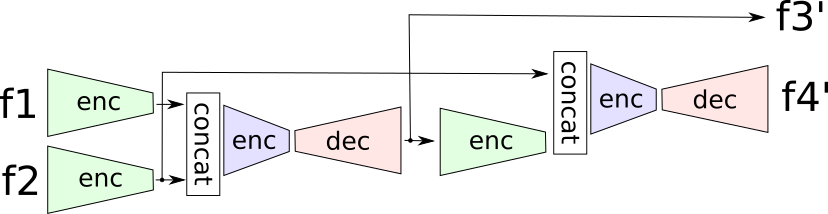we train this model on the sum of the L1 losses for $$f_3$$ and $$f_4$$ but continue to just use the v1 model section of the network for rollouts.

this teacher forcing gives a bit of help and things maybe improve a bit but it's still not great.

note: if you can see an RNN growing here you wouldn't be too far off. in hindsight maybe i should just stick an LSTM between the final encoder and the decoder stacks :/

 example rollout of v2 model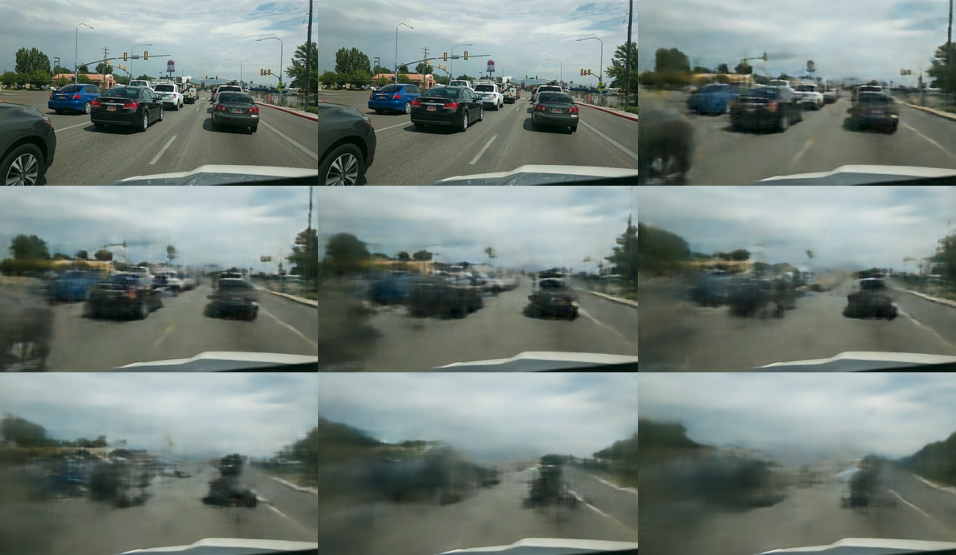## v3 model

the obvious next level up from L1 is to introduce a GAN loss. since the generators output is two sequential frames we make this the input for the discriminator.curiously this fails in a way i haven't seen before in a GAN... the output of G oscillates between two different modes. something weird to do with Gs output being two frames?

 example rollout of v3 model with oscillationif i can change D's input to be a single frame the oscillation goes away, but the results are still pretty crappy.

anyways, based on other models i've worked on that have components like this one (e.g. graspgan and bnn ) i know i'm at the point of tuning and tinkering but i've been distracted by something else in the short term... i'll come back to though one day...